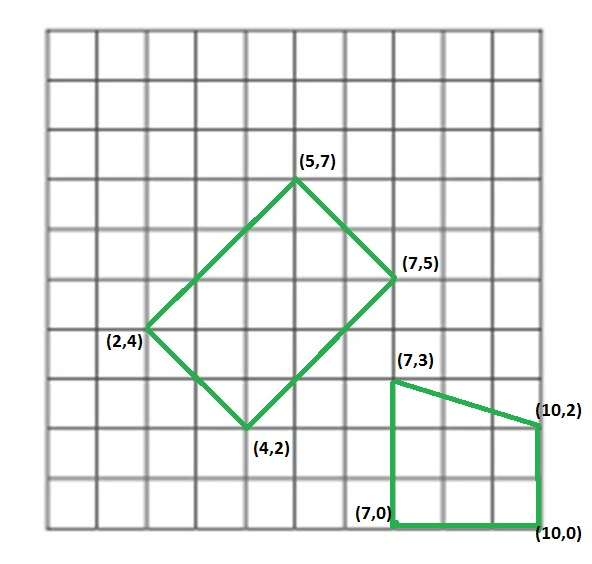Related Articles
Check if four segments form a rectangle
• Difficulty Level : Medium
• Last Updated : 04 Oct, 2018

We are given four segments as a pair of coordinates of their end points. We need to tell whether those four line segments make a rectangle or not.
Examples:

```Input : segments[] =  [(4, 2), (7, 5),
(2, 4), (4, 2),
(2, 4), (5, 7),
(5, 7), (7, 5)]
Output : Yes
Given these segment make a rectangle of length 3X2.

Input : segment[] = [(7, 0), (10, 0),
(7, 0), (7, 3),
(7, 3), (10, 2),
(10, 2), (10, 0)]
Output : Not
These segments do not make a rectangle.

Above examples are shown in below diagram.```

This problem is mainly an extension of How to check if given four points form a square

## Recommended: Please solve it on “PRACTICE ” first, before moving on to the solution.

We can solve this problem by using properties of a rectangle. First, we check total unique end points of segments, if count of these points is not equal to 4 then the line segment can’t make a rectangle. Then we check distances between all pair of points, there should be at most 3 different distances, one for diagonal and two for sides and at the end we will check the relation among these three distances, for line segments to make a rectangle these distance should satisfy Pythagorean relation because sides and diagonal of rectangle makes a right angle triangle. If they satisfy mentioned conditions then we will flag polygon made by line segment as rectangle otherwise not.

 `// C++ program to check whether it is possible ``// to make a rectangle from 4 segments ``#include  ``using` `namespace` `std; ``#define N 4 `` ` `// structure to represent a segment ``struct` `Segment ``{ ``    ``int` `ax, ay; ``    ``int` `bx, by; ``}; `` ` `// Utility method to return square of distance ``// between two points ``int` `getDis(pair<``int``, ``int``> a, pair<``int``, ``int``> b) ``{ ``    ``return` `(a.first - b.first)*(a.first - b.first) + ``        ``(a.second - b.second)*(a.second - b.second); ``} `` ` `// method returns true if line Segments make ``// a rectangle ``bool` `isPossibleRectangle(Segment segments[]) ``{ ``    ``set< pair<``int``, ``int``> > st; `` ` `    ``// putiing all end points in a set to ``    ``// count total unique points ``    ``for` `(``int` `i = 0; i < N; i++) ``    ``{ ``        ``st.insert(make_pair(segments[i].ax, segments[i].ay)); ``        ``st.insert(make_pair(segments[i].bx, segments[i].by)); ``    ``} `` ` `    ``// If total unique points are not 4, then ``    ``// they can't make a rectangle ``    ``if` `(st.size() != 4) ``        ``return` `false``; `` ` `    ``// dist will store unique 'square of distances' ``    ``set<``int``> dist; `` ` `    ``// calculating distance between all pair of ``    ``// end points of line segments ``    ``for` `(``auto` `it1=st.begin(); it1!=st.end(); it1++) ``        ``for` `(``auto` `it2=st.begin(); it2!=st.end(); it2++) ``            ``if` `(*it1 != *it2) ``                ``dist.insert(getDis(*it1, *it2)); `` ` `    ``// if total unique distance are more than 3, ``    ``// then line segment can't make a rectangle ``    ``if` `(dist.size() > 3) ``        ``return` `false``; `` ` `    ``// copying distance into array. Note that set maintains ``    ``// sorted order. ``    ``int` `distance; ``    ``int` `i = 0; ``    ``for` `(``auto` `it = dist.begin(); it != dist.end(); it++) ``        ``distance[i++] = *it; `` ` `    ``// If line seqments form a square ``    ``if` `(dist.size() == 2) ``    ``return` `(2*distance == distance); `` ` `    ``// distance of sides should satisfy pythagorean ``    ``// theorem ``    ``return` `(distance + distance == distance); ``} `` ` `// Driver code to test above methods ``int` `main() ``{ ``    ``Segment segments[] = ``    ``{ ``        ``{4, 2, 7, 5}, ``        ``{2, 4, 4, 2}, ``        ``{2, 4, 5, 7}, ``        ``{5, 7, 7, 5} ``    ``}; `` ` `    ``(isPossibleRectangle(segments))?cout << ``"Yes\n"``:cout << ``"No\n"``; ``} `

Output:

```Yes
```

This article is contributed by Utkarsh Trivedi. If you like GeeksforGeeks and would like to contribute, you can also write an article using contribute.geeksforgeeks.org or mail your article to contribute@geeksforgeeks.org. See your article appearing on the GeeksforGeeks main page and help other Geeks.